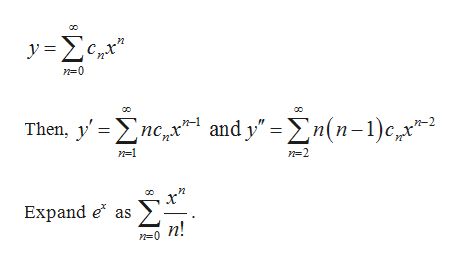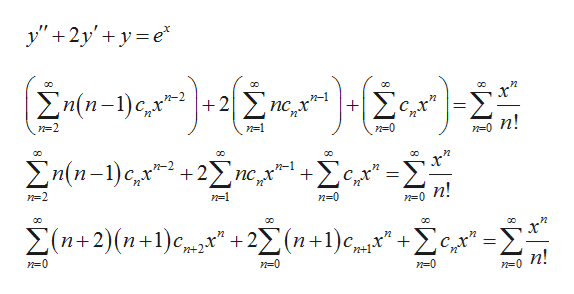# A point is an ordinary point of a non-homogeneous linear second order differential equation, y"+p(x)y'+q(x)y=f(x)is p, q, f are analytic at x0. Moreover, our theorem on the existence of power series solutions extends to such differential equations, ie, we can find power series solutions of non-homogeneous linear differential equations in the same manner as we have for homogeneous equations...almost. Find the series solution to the non-homogeneous initial value problem. Note: BEWARE THE THEOREM OF EQUIVALENT SERIES!!!Find the first five nonzero terms of the series solution to:y"+2y'+y=ex, y(0)=2, y'(0)=1

Question
105 views

A point is an ordinary point of a non-homogeneous linear second order differential equation,

y"+p(x)y'+q(x)y=f(x)

is p, q, f are analytic at x0. Moreover, our theorem on the existence of power series solutions extends to such differential equations, ie, we can find power series solutions of non-homogeneous linear differential equations in the same manner as we have for homogeneous equations...almost. Find the series solution to the non-homogeneous initial value problem. Note: BEWARE THE THEOREM OF EQUIVALENT SERIES!!!

Find the first five nonzero terms of the series solution to:

y"+2y'+y=ex, y(0)=2, y'(0)=1

check_circle

Step 1

Consider the differential equation:

Step 2

Let a power series solution of the differential equation behelp_outlineImage Transcriptionclosey n0 Then, yncnd y nn)c2 n-1 and y" -n(n-c,2 n=1 n 2 x Expand e as n! n-0 8 fullscreen
Step 3

Substitute y, y’ and y’’ in t...help_outlineImage Transcriptioncloseу"+2у'+ у 3е* оо со со Епи-1) с, х +2 пс х* + с п! n=1 n-0 п2 со х" сС оС со +Хсд -2 Хn(n-1) с, у* +22 nс n-1 пс х п n! n=2 и-1 n 0 СС оо со х -2 (п+1) с, + Хсд-X Хn+2)(n+1)с" +2) п! n-0 n-0 n-0 n=0 fullscreen

### Want to see the full answer?

See Solution

#### Want to see this answer and more?

Solutions are written by subject experts who are available 24/7. Questions are typically answered within 1 hour.*

See Solution
*Response times may vary by subject and question.
Tagged in

### Math